Semiconductor Diode - MSQ

# Semiconductor Diode - MSQ

Test Description

## 10 Questions MCQ Test Topic wise Tests for IIT JAM Physics | Semiconductor Diode - MSQ

Semiconductor Diode - MSQ for IIT JAM 2023 is part of Topic wise Tests for IIT JAM Physics preparation. The Semiconductor Diode - MSQ questions and answers have been prepared according to the IIT JAM exam syllabus.The Semiconductor Diode - MSQ MCQs are made for IIT JAM 2023 Exam. Find important definitions, questions, notes, meanings, examples, exercises, MCQs and online tests for Semiconductor Diode - MSQ below.
Solutions of Semiconductor Diode - MSQ questions in English are available as part of our Topic wise Tests for IIT JAM Physics for IIT JAM & Semiconductor Diode - MSQ solutions in Hindi for Topic wise Tests for IIT JAM Physics course. Download more important topics, notes, lectures and mock test series for IIT JAM Exam by signing up for free. Attempt Semiconductor Diode - MSQ | 10 questions in 45 minutes | Mock test for IIT JAM preparation | Free important questions MCQ to study Topic wise Tests for IIT JAM Physics for IIT JAM Exam | Download free PDF with solutions
 1 Crore+ students have signed up on EduRev. Have you?
*Multiple options can be correct
Semiconductor Diode - MSQ - Question 1

### The built-in potential of p-n junction diode is a function of.

Detailed Solution for Semiconductor Diode - MSQ - Question 1

Formation of potential of p-n junction diode depends on temperature, biased voltage and doping density.
The correct answers are: Temperature, Biased voltage, Doping density

*Multiple options can be correct
Semiconductor Diode - MSQ - Question 2

### An ideal switch is one which has :

Detailed Solution for Semiconductor Diode - MSQ - Question 2

Characteristics of any ideal switch is that it should offer zero resistance when ON and infinite resistance when OFF.
The correct answers are: Zero resistance when ON, Infinite resistance when OFF

*Multiple options can be correct
Semiconductor Diode - MSQ - Question 3

### For a full wave rectifier:

Detailed Solution for Semiconductor Diode - MSQ - Question 3

Full wave rectifier consists of two diodes each with different polarity ends which help it keep running both in positive and negative half. Average or dc value of load current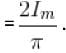The correct answers are: We need two diodes placed at opposite polarity, Ripple factor = 0.483, Maximum Rectification efficiency η = 81.1 %

*Multiple options can be correct
Semiconductor Diode - MSQ - Question 4

Which of the following statements is true in case of zener diode?

Detailed Solution for Semiconductor Diode - MSQ - Question 4

We know that zener diode has sharp breakdown voltage. It is always reverse biased and heavily doped diode. There is similarity between forward characteristic curve of a diode.
The correct answers are: A zener diode has sharp breakdown voltage, A zener diode is always reverse biased, The zener diode is a heavily doped diode

*Multiple options can be correct
Semiconductor Diode - MSQ - Question 5

Consider the following statements regarding the magnitude of barrier potential of a p- n junction. Which of them are true?

Detailed Solution for Semiconductor Diode - MSQ - Question 5

When a p-type semiconductor having holes as majority carrier is clamped with a ntype semiconductor having electron as majority carrier.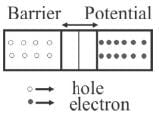Some electrons from n-side goes to p-side and some holes goes to n-side, thus creates a potential barrier, which
(i) depends on temperature
(ii) depends on fermi level
(iii) forbidden energy gap Eg
(iv) majority carriers
The correct answers are: It depends on difference between fermi levels on two sides of fermi level, It depends on forbidden energy-gap on two types of semiconductors, It depends on impurity concentration in p and n-type semiconductors

*Multiple options can be correct
Semiconductor Diode - MSQ - Question 6

Which of the following devices is forward bias in its normal mode of operation.

Detailed Solution for Semiconductor Diode - MSQ - Question 6

Zener diode and Avalanche photodiode operates only in reverse bias condition. The correct answers are: Solar cell, LASER diode

*Multiple options can be correct
Semiconductor Diode - MSQ - Question 7

If the barrier potential change with biasing of a p-n junction diode, then the barrier potential will.

Detailed Solution for Semiconductor Diode - MSQ - Question 7

For a p-n junction diode, barrier potential decreases with forward and increases with reverse biasing.
The correct answers are: increase with reverse bias, decrease with forward bias

*Multiple options can be correct
Semiconductor Diode - MSQ - Question 8

For an intrinsic semiconductor,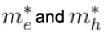are respectively, the effective masses of electrons and holes near the corresponding band edges. At a finite temperature, the position of fermi level:

Detailed Solution for Semiconductor Diode - MSQ - Question 8

The finite temperature, the position of fermi level would depend on bothThe correct answers are: depends on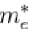, depends on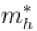*Multiple options can be correct
Semiconductor Diode - MSQ - Question 9

Which of the following statements are true for a clamper?

Detailed Solution for Semiconductor Diode - MSQ - Question 9

Any clamper consists of a diode, resistor and capacitor. It does not require any inductor. It does not change the appearance but only shifts the dc level of any given signal.
The correct answers are: does not change appearance of input signal, shifts dc level of the waveform

*Multiple options can be correct
Semiconductor Diode - MSQ - Question 10

Which of the following electronic devices are used to make a clamper?

Detailed Solution for Semiconductor Diode - MSQ - Question 10

A normal clamper consists of a diode, a resistor and a capacitor that shifts a waveform to a different dc level without changing the appearance of the applied signal.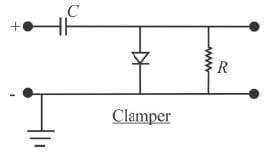The correct answers are: Capacitor, Diode, Resistor

## Topic wise Tests for IIT JAM Physics

217 tests
Information about Semiconductor Diode - MSQ Page
In this test you can find the Exam questions for Semiconductor Diode - MSQ solved & explained in the simplest way possible. Besides giving Questions and answers for Semiconductor Diode - MSQ, EduRev gives you an ample number of Online tests for practice

## Topic wise Tests for IIT JAM Physics

217 tests(Scan QR code)